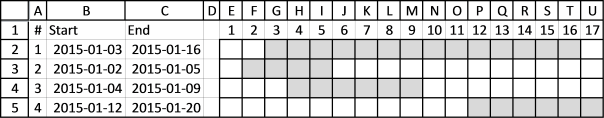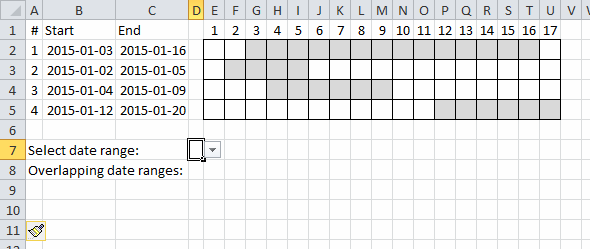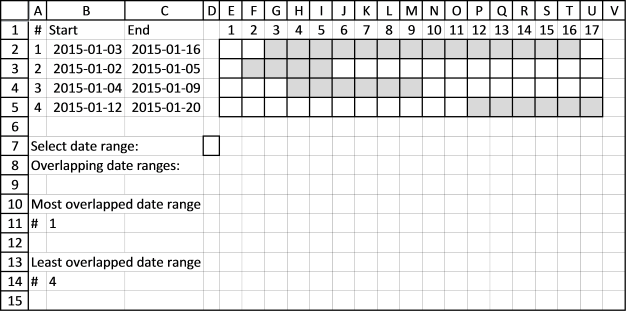Author: Oscar Cronquist Article last updated on July 27, 2017

Today's blog post is about date ranges, the techniques demonstrated here can also be applied to time or other numerical ranges. The MMULT function is a great excel function, it allows you to do really amazing calculations with date ranges. Yes, I have said that before.

For simplicity, in this example there are only 4 date ranges in column B and C and you can see their length in the chart to the right.Let's begin with a simple formula, it will be helpful for you if you understand this one. It calculates the number of date ranges that overlaps the first date range:

=SUMPRODUCT((B2<=C2:C5)*(C2>=B2:B5))-1

It returns 3 overlapping date ranges, which is correct. Range 2, 3 and 4 overlap range 1.

Find overlapping date ranges.

### Calculate all ranges

How do we calculate the number of overlapped ranges for all ranges in one formula?

=MMULT((B2:B5<=TRANSPOSE(C2:C5))*(C2:C5>=TRANSPOSE(B2:B5)),B2:B5^0)-1

This array formula returns an array: {3;2;2;1}. The first date range has 3 overlapping ranges, described above. The second has 2, range 1 and 3 overlaps range 2. The third has 2 overlapping ranges, range 1 and 2. The fourth range has 1 overlapping date range, range 1.

In the animated picture below the drop down list in cell D7 allows you to select a date range, cell D8 tells you how many overlapping date ranges the selected date range has.I applied conditional formatting to easily spot the selected date range.

Array formula in cell D8:

=IF(D7="","",INDEX(MMULT((B2:B5<=TRANSPOSE(C2:C5))*(C2:C5>=TRANSPOSE(B2:B5)),B2:B5^0)-1,D7))

### Find most overlapped date rangeUsing this technique we can now construct a formula that finds the most overlapped date range, array formula in cell B11:

=MATCH(MAX(MMULT((B2:B5<=TRANSPOSE(C2:C5))*(C2:C5>=TRANSPOSE(B2:B5)),B2:B5^0)-1),MMULT((B2:B5<=TRANSPOSE(C2:C5))*(C2:C5>=TRANSPOSE(B2:B5)),B2:B5^0)-1,0)

Here is the formula for the least overlapped date range, cell B14:

=MATCH(MIN(MMULT((B2:B5<=TRANSPOSE(C2:C5))*(C2:C5>=TRANSPOSE(B2:B5)),B2:B5^0)-1),MMULT((B2:B5<=TRANSPOSE(C2:C5))*(C2:C5>=TRANSPOSE(B2:B5)),B2:B5^0)-1,0)

Want to know more about the excel functions used in the formulas above?
SUMPRODUCT, MMULT, INDEX, MATCH